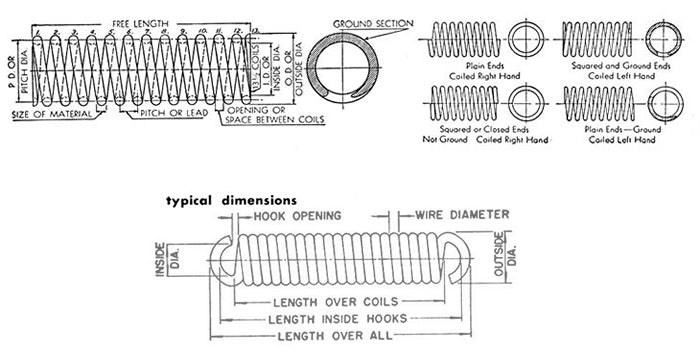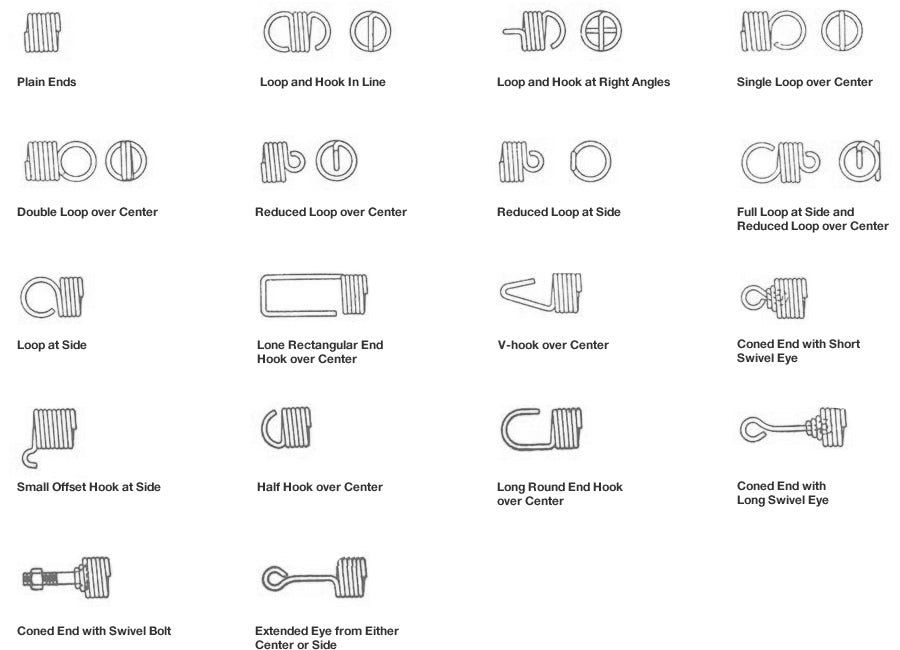## Technical Information## The number of coils

The number of coils in tension springs varies with commercial production as follows:
 Number of Coils Tolerance 3 to 5 ±20° 6 to 8 ±30° 9 to 12 ±40°

For each additional coil, add 1½° per coil. Springs can be built to closer tolerances by trimming after coiling, with additional cost.

The load tolerances for tension springs are listed in the above table. For example of how to use these, let us take a spring of the following dimensions:
Free Length 4.050”; Mean Dia. .390”; Wire Dia. .048” to give a load of 3.5lb when extended .900”.Where the line 4.5 intersects with the column of wire dia. of .048” we get a figure of ±10.1%.

This is the load tolerance of the above example.

Convert 10.1% to 3.5 lb. which gives a tolerance of 5.6 oz. at .900” deflection.

## Type of Ends